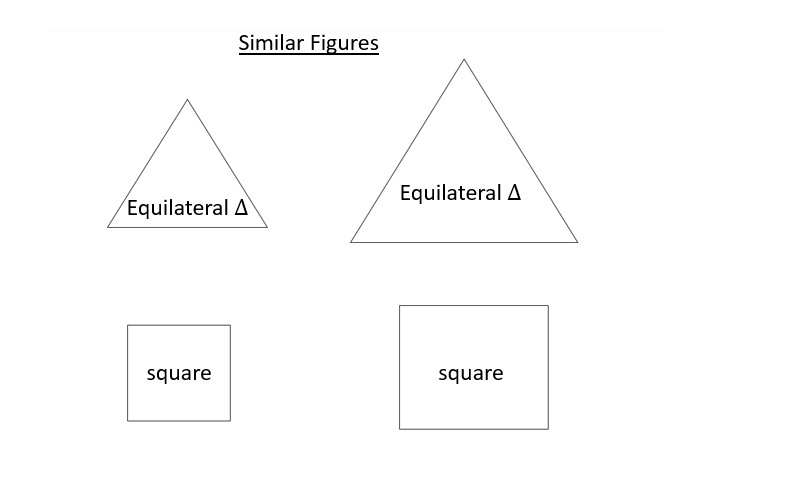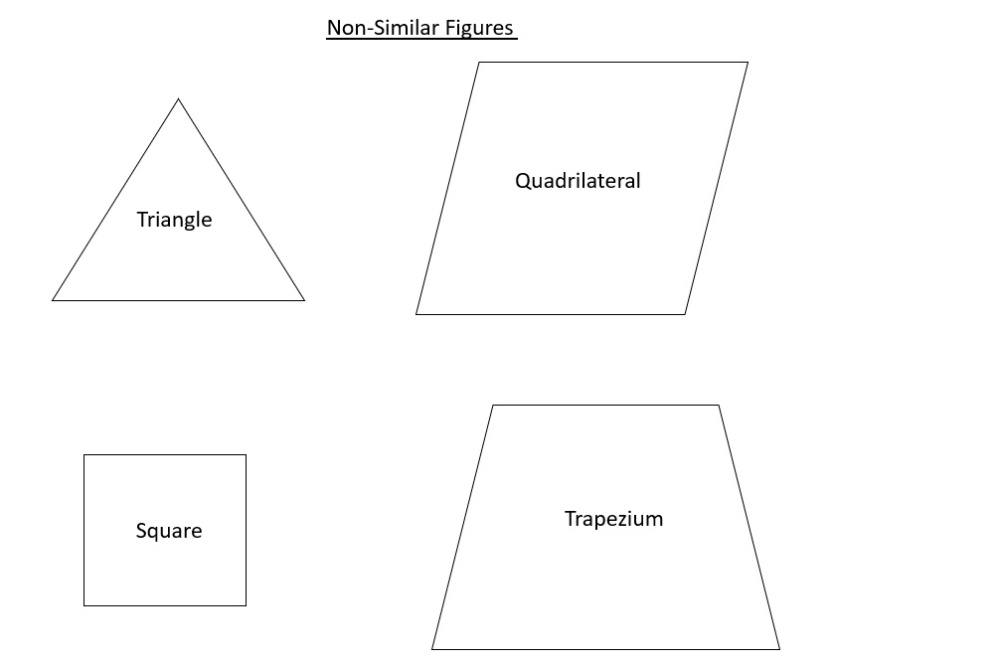Home/Class 10 Maths Chapter List/4. Triangles/

# Give two different examples of pair of (i) similar figures.(ii) non-similar figures.

Give two different examples of pair of
(i) similar figures.
(ii)  non-similar figures.Speed
00:00
03:45## QuestionMathsClass 10

Give two different examples of pair of
(i) similar figures.
(ii)  non-similar figures.

See the solution below.
4.64.6## Solution

$$(i)$$ (a)Pair of equilateral triangles are similar figures.
(b) Pair of squares are similar figures.$$(ii)$$ (a) A triangle and a quadrilateral form a pair of non-similar figures.
(b) A square and a trapezium form a pair of non-similar figures.